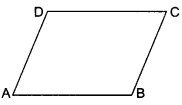# DAV Class 8 Maths Chapter 10 Brain Teasers Solutions

The DAV Class 8 Maths Book Solutions and DAV Class 8 Maths Chapter 1 Brain Teasers Solutions of Parallel Lines offer comprehensive answers to textbook questions.

## DAV Class 8 Maths Ch 10 Brain Teasers Solutions

Question 1.
Tick (✓) the correct option.
(i) A transversal intersects two lines. Which condition would always make the two lines parallel?
(а) Vertically opposite angles are equal.
(b) Interior angles on the same side of the transversal are complementary.
(c) Alternate interior angles are equal.
(d) Corresponding angles are supplementary.
(c) Alternate interior angles are equal.

(ii) Based on the diagram given below, which of the following statements is true?(a) a ∥ b
(b) a ∥ c
(c) b ∥ c
(d) d ∥ e
(ii) x + 110° = 180° (Linear pair)
⇒ x = 70°
y + 60° = 180° (Linear pair)
⇒ y = 120°
z + 120° = 180° (Linear pair)
⇒ z = 60°Lines a and b are not parallel because alternate angles x = 70° and 60° are not equal.
Lines a and c are not parallel because corresponding angles 110° and 115° are not equal.
Lines b and c are not parallel because corresponding angles y = 120° and 115° are not equal.
Lines d and e are parallel because alternate angles z = 60° and 60° are equal. Thus, option (d) is the correct answer.

(iii) In the diagram given below, transversal TU intersects PQ and RS at V and W, respectively. For which value of x is PQ ∥ RS?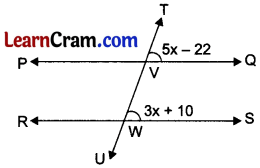(a) 6
(b) 16
(c) 24
(d) 28
Lines PQ ∥ RS when corresponding angles are equal.
∴ 5x – 22 = 3x + 10
⇒ 5x-3x = 22 + 10
⇒ 2x = 32 ⇒ x = 16
Thus, option (b) is the correct answer.

(iv) Peach Street and Cherry Street are parallel. Apple Street intersects them, as shown below:If m∠1 = (2x + 36)°, m∠2 = (7x – 9)°, what is m∠1
(a) 9°
(b) 17°
(c) 54°
(d) 70°
Let Peach street and Cherry street be parallel lines.
Then, m ∠1 – m∠3 (Vertically opposite angles).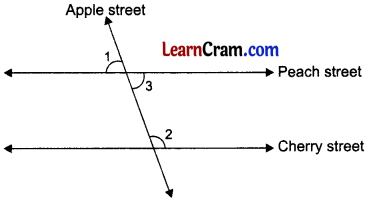⇒ (2x + 36)° = m∠3 or m∠3 = (2x + 36)°
Also, m∠2 + m∠3 = 180° (Interior angles on the same side of transversal)
⇒ 7x – 9° + 2x + 36° = 180
⇒ 9x + 27° = 180°
⇒ 9x = 180° – 27 = 153°
⇒ x = 17°
Thus, option (b) is the correct answer.

(v) In the diagram given below, lines n and m are cut by transversals p and q?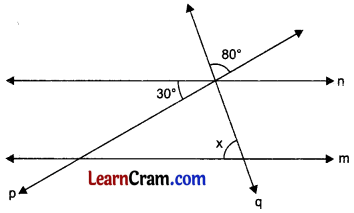What value of x would make lines n and m parallel?
(a) 110°
(b) 80°
(c) 70°
(d) 50°
Let lines n and m be parallel lines.Then, y = 80° (Vertically opposite angles)
Also, (y + 30)° + x = 180° (Interior angles on the same side of the transversal q)
⇒ (80° + 30°) + x = 180°
⇒ x = 180° – 110°
⇒ x = 70°
Thus, option (c) is the correct answer.Question 1B.
(i) If $$\overline{\mathrm{AB}} \| \overline{\mathrm{DE}}$$, find ∠ACD.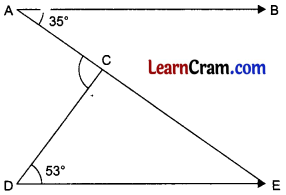We have AB ∥ DE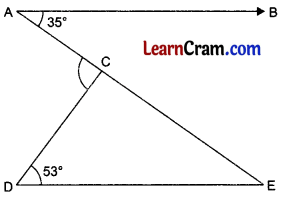∠BAC = ∠CED
(Alternate angles)
⇒ ∠CED = 35° (∵ ∠BAC = 35°)
In ACDE, ∠ACD = ∠CDE + ∠CED
(Exterior angle property)
⇒ ∠ACD = 53° + 35°
⇒ ∠ACD = 88°

(ii) In the diagram given below, $$\overline{\mathrm{PQ}} \| \overline{\mathrm{OL}}$$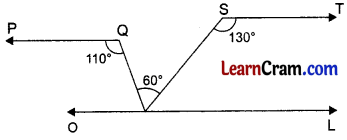Is $$\overline{\mathrm{OL}} \| \overline{\mathrm{ST}}$$ ? Give reasons.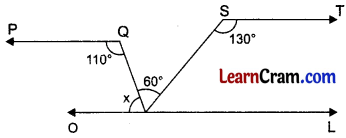Given that $$\overline{\mathrm{PQ}} \| \overline{\mathrm{OL}}$$
⇒ x + 110° = 180°
(Interior angles on the same side of transversal)
⇒ x = 180° -110° = 70°
Now, x + 60° = 70° + 60° = 130°.
Clearly, (x + 60°) = ∠S = 130° and these are alternate angles.
Hence, lines $$\mathrm{OL} \| \mathrm{ST}$$.

(iii) If $$\overline{\mathrm{AB}} \| \overline{\mathrm{PQ}}$$, find y.Through O, draw a line parallel to both AB and PQ.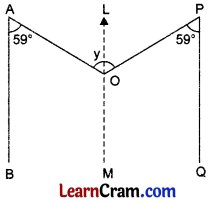Then, ∠AOL = ∠BAO = 59° (Alternate angles)
and ∠POL = ∠QPO = 59° (Alternate angles)
Now, y = ∠AOL + ∠POL = 59° + 59° = 118°.

(iv) If $$\overline{\mathrm{AB}}\|\overline{\mathrm{EF}}, \overline{\mathrm{DE}}\| \overline{\mathrm{BC}}, \overline{\mathrm{AC}} \| \overline{\mathrm{DF}}$$, find x and y.If AB ∥ EF and DE is a transversal,
then ∠E + 110° = 180° (Interior angles on the same side of DE)
⇒ ∠E = 180°-110°
⇒ ∠E = 70°Now, if DE ∥ BC and EF is a transversal,
then x + ∠E = 180° (Interior angles on the same side of EF)
⇒ x + 70° = 180°
⇒ x = 180°-70° =» *= 110°
Finally, if AC ∥ DF and DE is a transversal, then y + 60° = 180° (Interior angles on the
same side of DE)
⇒ y = 180° – 60° = 120°
⇒ y = 120°
Thus, x = 110° andy = 120°.

(v) For what value of x, is l ∥m?Let lines l and m be parallel.Then, ∠1 = 45° (Vertically opposite angles)
Also, ∠1 + (5x + 35)° = 180°
(Interior angles on the same side of transversal t)
⇒ 45° + 5x + 35° = 180°
⇒ 5x + 80° = 180°
⇒ 5x = 180° – 80° = 100°
⇒ x = 20°Question 2.
In the given figure, l ∥ m ∥ n and p ∥ q ∥ r. If ∠1 = 85°, find ∠x, ∠y, ∠z, ∠t, ∠s and ∠u.Here l ∥ m ∥ n and p ∥ q ∥ r.
∴ ∠1 = ∠x (Alternate angles)
85° = ∠x
∴ ∠x = 85°
∠x = ∠z (Corresponding angles)
85° = ∠z
∴ ∠z = 85°

∠1 = ∠s (Corresponding angles)
85° = ∠s
∴ ∠s = 85
∠s + ∠u = 180° (Linear pairs)
85° + ∠u = 180°
∴ ∠u = 180° – 85° = 95°

∠u + ∠t = 180° (Sum of interior angles)
⇒ 95° + ∠t = 180°
⇒ ∠t = 180° – 95° = 85°
⇒ ∠y + ∠t = 180° (Sum of interior angles)
⇒ ∠y + 85° = 180°
∠y = 180° – 85° = 95°
Hence, ∠x = 85°, ∠y = 95°, ∠z = 85°, ∠u = 95°, ∠t = 85°, ∠s = 85°.

Question 3.
Draw a line segment AB = 7.6 cm. Without constructing parallel lines at A and B, find three points P, Q and R on $$\overline{\mathrm{AB}}$$ such that $$\overline{\mathrm{AP}}=\overline{\mathrm{PQ}}=\overline{\mathrm{QR}}=\overline{\mathrm{RB}}$$
Steps of Construction:
1. Draw AB = 7.6 cm.
2. With the help of compass, draw a right bisector of $$\overline{\mathrm{AB}}$$ at Q.
3. Draw the right bisectors of $$\overline{\mathrm{AQ}}$$ at P and $$\overline{\mathrm{QB}}$$ at R.
4. $$\overline{\mathrm{AP}}, \overline{\mathrm{PQ}}, \overline{\mathrm{QR}}$$ and $$\overline{\mathrm{RB}}$$ are the required four equal divisions of $$\overline{\mathrm{AB}}$$.

Question 4.
In the given figure, name the edges of the following cube which are parallel to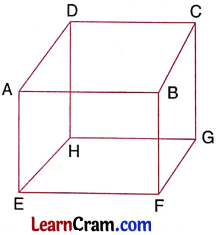(i) $$\overline{\mathrm{AB}}$$
$$\overline{\mathrm{DC}}, \overline{\mathrm{HG}}, \overline{\mathrm{EF}}$$

(ii) $$\overline{\mathrm{EH}}$$
$$\overline{\mathrm{FG}}, \overline{\mathrm{AD}}, \overline{\mathrm{BC}}$$

(iii) $$\overline{\mathrm{AE}}$$
$$\overline{\mathrm{DH}}, \overline{\mathrm{BF}}, \overline{\mathrm{CG}}$$

(iv) What is the point of intersection of $$\overline{\mathrm{AE}}$$ and $$\overline{\mathrm{AB}}$$?
A

(v) Are the edges $$\overline{\mathrm{AB}}$$ and $$\overline{\mathrm{DH}}$$ parallel to each other? Why?
$$\overline{\mathrm{AB}}$$ is not parallel to $$\overline{\mathrm{DH}}$$ because they are the edges of different faces and perpendicular to each other.

(vi) Are edges $$\overline{\mathrm{EF}}$$ and $$\overline{\mathrm{BC}}$$ parallel?
$$\overline{\mathrm{DH}}$$ is not parallel to $$\overline{\mathrm{BC}}$$ because they are different faces perpendicular to each other.

### DAV Class 8 Maths Chapter 10 HOTS

Question 1.
In the diagram given below, find the angles x, y and z.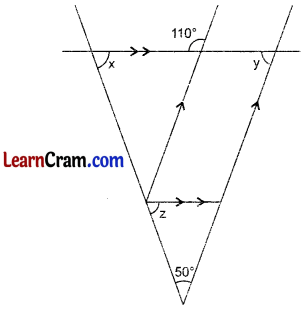a + 110° = 180° (Linear pair)
⇒ a = 180° – 110° = 70°
⇒ a = 70°
Lines ED ∥ FB and AB is a transversal.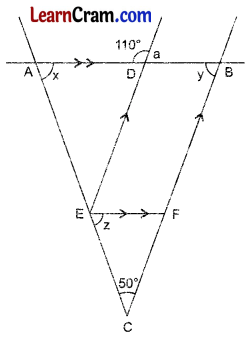Then, a = y (Alternate angles)
⇒ y = 70° (∵ a = 70°)
Now, in ΔABC,
x + 50° + y = 180°
⇒ x + 50° + 70° = 180°
⇒ x + 120° = 180°
⇒ x = 60°
Again, lines AD ∥ EF and AE is a transversal.
Then, x = z (Corresponding angles)
⇒ z = 60°
Thus, x = 60°, y = 70°, z = 60°.

Question 2.
In the figure, l ∥ m and ‘t’ is the transversal. The angle bisectors of interior angles intersect at P and Q. Find the value of angles x and y.Since interior angles on the same side of the transversal are supplementary.
∴ ∠EAB + ∠TBA = 180°
⇒ $$\frac{1}{2}$$∠EAB + $$\frac{1}{2}$$∠TBA = $$\frac{1}{2}$$ × 180°
AQ and BQ are bisectors of ∠EAB and ∠TBA, respectively
∠1 = ∠EAB and ∠2 = ∠TBA
From (i) and (ii), we get
∠1 + ∠2 = 90° …(iii)

In MEQ, we have 4
∠1 + ∠2 + x = 180°
90 + x = 180° [Using (iii)]
x = 180° – 90°
x = 90°
Similarly, ∠APB = 90°
Now, ∠APB = y (Vertically opposite angles)
∴ y = 900
Thus, x = 90°, y = 90°.

### DAV Class 8 Maths Chapter 10 Enrichment Questions

Question 1.
You are given three platforms parallel to each other. Help Kitty to reach from Barrel 1 to Barrel 2 by finding all the missing angles.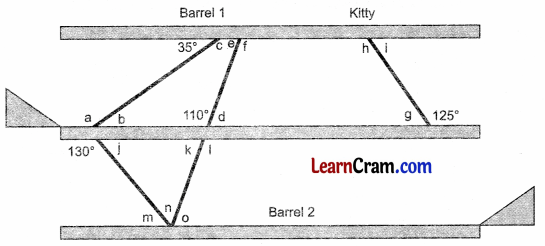All the three platforms are parallel to each other.
Then, 35° + c = 180° (Linear pair)
⇒ c = 180° – 35° = 145° ∴ c = 145 …(1)
b = 35° …(2) (Alternate angles)
⇒ a = 145° ….(3) [a and c are alternate angles]
d + 110° = 180° (Linear pair)
⇒ d = 180° – 110° = 70°
⇒ d = 70 …(4)
e = d (Alternate angles)

⇒ e = 70° …(5) [Using (4)]
f = 110° ……..(6) (Alternate angles)
g + 125° = 180° (Linear pair)
g = 180° – 125°
⇒ g = 55° …(7)
g = i (Alternate angles)

⇒ i = 55° …(8) [Using (7)]
h = 125° …….(9) (Alternate angles)
j + 130° = 180° (Linear pair)
⇒ j = 50° …(10)
j = m (Alternate angles)

⇒ m = 50° …(11) [Using (10]
k = d (Vertically opposite angles)
⇒ k = 70° …(12) [Using (4)]
k + l = 180° (Linear pair)
⇒ 70° + l = 180°
⇒ l = 110 …(13)
k = o (Alternate angles)

⇒ o = 70° …(14)[Using 12]
m + n + o = 180° (Straight angles)
⇒ 50° + n + 70° = 180° [Using (11) and (14)]
⇒ n + 120° = 180° …(15)
Thus, a = 145°, b = 35°, c = 145°, d = 70°, e = 70 °, f= 110°, g = 55°, h = 125°, i = 55°, j = 50°, k = 70°, l = 110°, m = 50°, n = 60°, o = 70°.

Question 1.
In the given figure, ∠1 = 135°, find ∠x, ∠y, ∠z, ∠t and ∠u.Here l ∥ m ∥ n and p ∥ q
∠1 = ∠u [Alternate angles]
∠1 = 135° (Given)
∴ ∠u = 135°
∠u = ∠x
∴∠x = 135°

∠x + ∠y = 180°
135° + ∠y = 180°
∠y = 180° – 135° = 45°
∠y = ∠t [Corresponding angles]
45° = ∠t
∴ ∠t = 45°
∠t = ∠z [Corresponding angles]
45° = ∠z
∴ ∠z = 45°
Hence, ∠x = 135°, ∠y = 45°, ∠z = 45° ∠t = 45° ∠u = 135°Question 2.
In the given figure, ABCD is a parallelogram. CD is f P produced to E. If ∠1 = 75°, find all the angles of the parallelogram ABCD.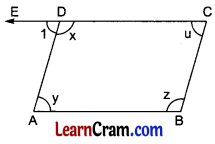∠1 = 75° [Given]
∠1 + ∠x = 180° [Linear pairs]
75° + ∠x = 180°
∴ ∠x = 180° – 75° = 105°
∠1 = ∠y [Alternate angles]
75° = ∠y
∠y = 75°
∠1 = ∠u [Corresponding angles]
75° = ∠u
∴ ∠u = 45°
∠u + ∠z = 180° [Sum of interior angles]
75° + ∠z = 180°
∠z = 180° – 75° = 105°
Hence, ∠x = 105°, ∠y = 75°, ∠z = 105° and ∠u = 75°.

Question 3.
Divide a line segment $$\overline{\mathrm{AB}}$$ = 6 cm into 5 equal parts.
Steps of Construction:
1. Draw $$\overline{\mathrm{AB}}$$ = 6 cm.
2. Draw $$\overrightarrow{\mathrm{AP}}$$ parallel to $$\overrightarrow{\mathrm{BQ}}$$ at A and B respectively making equal acute angles on opposite sides.
3. Mark A1; A2, A3, A4, A5 on $$\overrightarrow{\mathrm{AP}}$$ and Bx, B2, B3, B4 and B5 on $$\overrightarrow{\mathrm{BQ}}$$.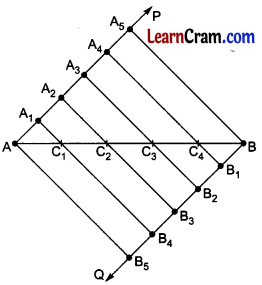4. Join AB5, A1B4, A2B3, A3B2, A3B2, A4B1 and A5B which cut AB at C2, C2, C3 and C4.
5. Hence $$\overline{\mathrm{AC}_1}, \overline{\mathrm{C}_1 \mathrm{C}_2}, \overline{\mathrm{C}_2 \mathrm{C}_3}, \overline{\mathrm{C}_3 \mathrm{C}_4}$$ and C4B are equal parts of latex]\overline{\mathrm{AB}}[/latex].

Question 4.
Divide a line segment of length 5.5 cm in the ratio 2 : 3 internally.
Steps of Construction:
1. Draw AB = 5.5 cm.
2. Draw $$\overrightarrow{\mathrm{AP}} \| \overrightarrow{\mathrm{BQ}}$$ at A and B respectively making acute angles on opposite sides.
3. With the help of compass, mark A1, A2, A3, A4, A5 on $$\overrightarrow{\mathrm{AP}}$$ and B1, B2, B3, B4, B5 on $$\overrightarrow{\mathrm{AP}}$$ at equal distances.4. Join AB5 and BA5.
5. Join A2 to B3 which cuts $$\overline{\mathrm{AB}}$$ at C.
6. Hence, C is the required point which divides $$\overline{\mathrm{AB}}$$ in the ratio 2:3.

Question 5.
Draw a line segment AB = 5 cm. Divide it in the ratio 2 : 3 externally.
Steps of Construction:
1. Draw $$\overline{\mathrm{AB}}$$ = 5 cm.
2. Draw $$\overrightarrow{\mathrm{AP}}$$ at A making an acute angle.
3. Divide $$\overrightarrow{\mathrm{AP}}$$ in 3 equal parts AA1, A1A2 and A2A3.4. 3 – 2 = 1, so join A1 to B.
5. Draw a line from A3 parallel to A1B to meet $$\overline{\mathrm{AB}}$$ produced at C.
6. Hence the required ratio is $$\overrightarrow{\mathrm{AP}}$$ =2:3 externally.Question 6.
Draw a line segment $$\overline{\mathrm{AB}}$$ = 6 cm and divide it in the ratio 4 : 2 internally.
Steps of Construction:
1. Draw AB = 6 cm.
2. Draw AP ∥ BQ at A and B respectively making acute angle on opposite sides.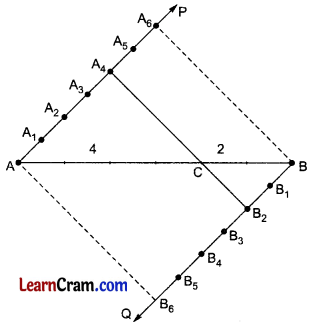3. Mark A1, A2 A3, A4, A5, A6 and B1, B2, B3, B4, B5 and B6 on $$\overrightarrow{\mathrm{AP}}$$ and $$\overrightarrow{\mathrm{AP}}$$ $$\overrightarrow{\mathrm{AQ}}$$.
4. Join A4 to B2 which cuts $$\overline{\mathrm{AB}}$$ at C which is the required point dividing $$\overline{\mathrm{AB}}$$ in the ratio 4 : 2.

Question 7.
In the given figure, l ∥ m and n is a transversal. If ∠1 = 70°,then the measure of ∠2 is(a) 70°
(b) 110°
(c) 90°
(d) 120°
∠1 = ∠3 = 70°
∠2 + ∠3 = 180°
∠2 + 70° = 180°
⇒ ∠2 = 180° – 70° = 110°
Hence, (b) is correct.

Question 8.
In the given figure, if l ∥ m ∥ n, find the measure of ∠x and ∠y.l ∥ m ∥ n (Given)
AB and BC are transversals.
∠x + 105° = 180° (Sum of interior angles)
∠x = 180° – 105° = 75°
∠y = 35° [Alternate angles]
Hence, ∠x = 75° and ∠y = 35°

Question 9.
In the given figure, l ∥ m and n is a transversal. If ∠1 = (5x + 25)° and ∠2 = (15x – 35)°, then find the value of x and hence find the measure of ∠3.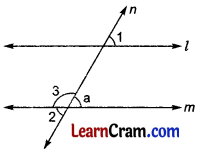∠1 = ∠a
But ∠a = ∠2
∴ ∠1 = ∠2
5x + 25 = 15x – 35
⇒ 5x – 15x = – 35 – 25
– 10x = – 60
∴ x = 6
∴ ∠2 = 15x – 35 = 15 × 6 – 35 = 90 – 35 = 55
∠2 + ∠3 = 180°
⇒ 55° + ∠3 = 180°
⇒ ∠3 = 180° – 55° = 125°
Hence, x = 6 and ∠3 = 125°.

Multiple Choice Questions:

Question 1.
In the given figure, the value of ∠a: is(a) 120°
(b) 130°
(c) 140°
(d) 115°
(b) 130°

Question 2.
∠A and ∠B are supplementary angles. If ∠A = 30°, then ∠B is:
(a) 150°
(b) 120°
(c) 110°
(d) 115°
(a) 150°

Question 3.
In the given figure, the values of ∠x and ∠y are,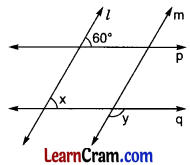(a) 60° and 120°
(6) 50° and 110°
(c) 60° and 105°
(d) none of these.
(a) 60° and 120°

Question 4.
If P divides AB in the ratio 3 : 4, then the ratio of AP and AB is
(a) 7 : 3
(b) 5:7
(c) 3:7
(d) 4:7